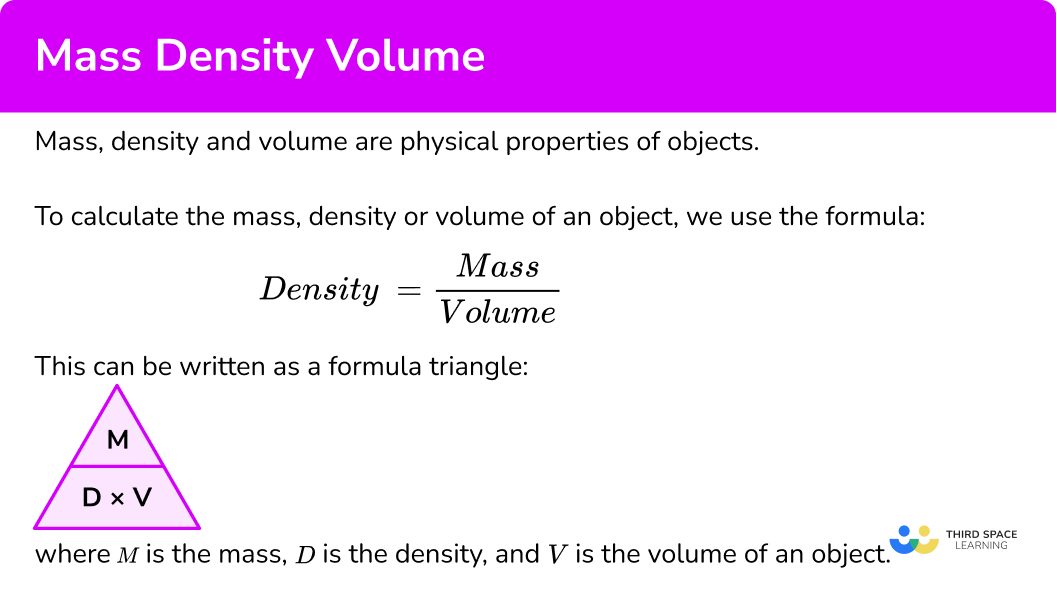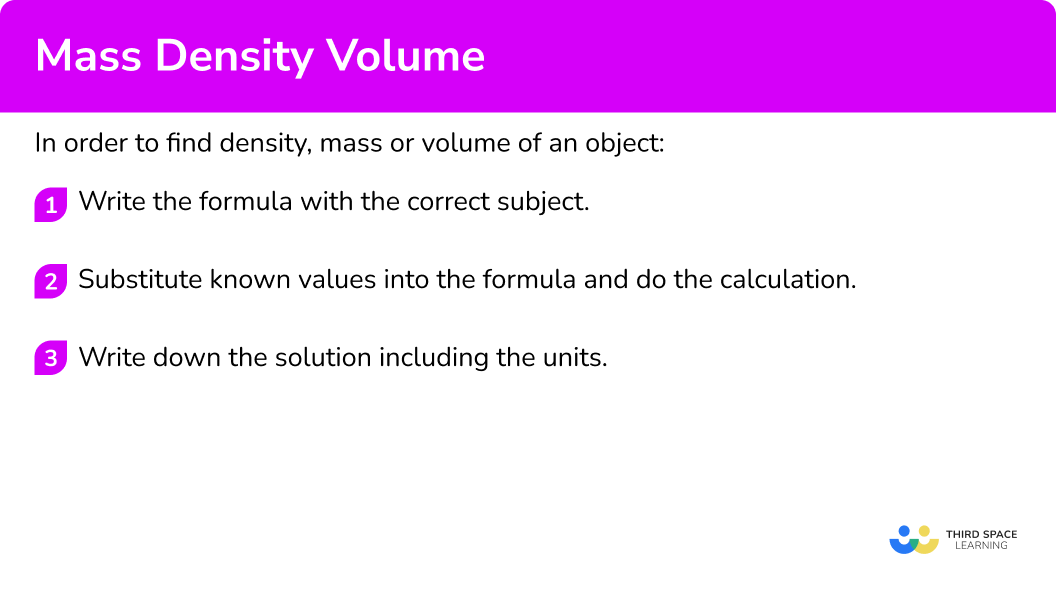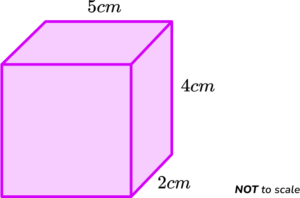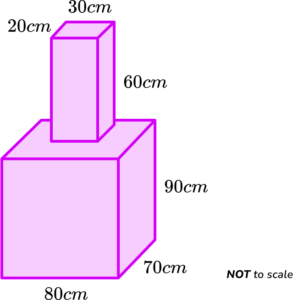# Mass Density Volume

Here we will learn about mass, density and volume, including what they are and how they are related to each other.

There are also mass density volume worksheets based on Edexcel, AQA and OCR exam questions, along with further guidance on where to go next if you’re still stuck.

## What is mass density volume?

Mass, density and volume are physical properties of objects.

• Mass

Mass is the measurement of the amount of matter there is in an object. The units of mass are usually kilograms (kg) or grams (g). The kilogram is the SI unit (the International System of Units) for mass.

• Volume

Volume is a measure of how much space an object takes up. The volume of a substance is often measured in cubic centimetres (cm^{3}). The derived SI unit for volume is the cubic metre (m^{3}).

• Density

Density is a compound measure made from the ratio of mass and volume. Density is a measure of the amount of matter there is per unit volume. The SI unit for density is kilograms per cubic metre (kg/m^{3}).

Imagine there are two objects the same size. The first object has high density, the second object has a lower density. The object with high density would feel heavier than the object with the lower density.

You could try this with two water bottles. Fill one with water and fill the other with air.

The density of water is approximately 1000 \ kg/{m}^{3}.

The density of air is approximately 1.225 \ kg/{m}^{3}.

The density of water is greater than the density of air, so the bottle filled with water is heavier.

### What is mass density volume?### Density

To calculate the mass, density or volume of an object, we use the formula:

\text{Density}=\frac{\text{Mass}}{\text{Volume}}

This can be written as a formula triangle:

where M is the mass, D is the density, and V is the volume of an object.

Using this triangle, we can show:

• M=D\times{V}
• D=M\div{V}
• V=M\div{D}

Note: The relative density, or specific gravity is the relationship between the density of a substance in relation to the density of another referenced substance.

For example, water at 4^{o}C has a density of 1kg/l. Liquid mercury has a density of 13.6kg/l and so its specific gravity is 13.6 as 13.6\div{1}=13.6. As both units are in kg/l, specific gravity has no units.

## How to find the density, mass or volume

In order to find density, mass or volume:

1. Write the formula with the correct subject.
2. Substitute known values into the formula and do the calculation.
3. Write down the solution including the units.

### Explain how to find the density, mass or volume### Related lessons oncompound measures

Mass density volume is part of our series of lessons to support revision on compound measures. You may find it helpful to start with the main compound measures lesson for a summary of what to expect, or use the step by step guides below for further detail on individual topics. Other lessons in this series include:

## Density mass volume examples

### Example 1: calculating the density (g/cm3)

A sphere is made from gold. It has volume 3 \ cm^3 and mass 60 \ g. Calculate the density of the object.

1. Write the formula with the correct subject.

To calculate the density, we would need to divide the mass of the object by the volume of the object.

\text{Density}=\frac{\text{Mass}}{\text{Volume}}

2Substitute known values into the formula and do the calculation.

\text{Density}=\frac{\text{Mass}}{\text{Volume}}=\frac{60}{3}=20

3Write down the solution including the units.

The density of the object is 20\text{ g/cm}^{3} .

### Example 2: calculating the density (kg/m3)

An object is made from steel. It has a volume of 25 \ m^3 and a mass of 425 \ kg. Calculate the density of the object.

To calculate the density, we need to divide the mass of the object by the volume of the object.

\text{Density}=\frac{\text{Mass}}{\text{Volume}}

\text{Density}=\frac{\text{Mass}}{\text{Volume}}=\frac{425}{25}=17

The density of the object made of steel is 17 \ kg/m^{3}.

### Example 3: calculating the volume (cm3)

The mass of an object is 600 \ g. The density of the substance which the object is made from is 3.2 \ g/cm^{3}. Calculate the volume of the object.

To calculate the volume, we need to divide the mass of the object by the density of the substance.

\text{Volume}=\frac{\text{Mass}}{\text{Density}}

\text{Volume}=\frac{\text{Mass}}{\text{Density}}=\frac{600}{3.2}=187.5

The volume of the object is 187.5 \ cm^{3}.

### Example 4: calculating the volume (m3)

The mass of an object is 1500 \ g. The density of the substance which the object is made from is 60 \ kg/m^{3}. Calculate the volume of the object.

To calculate the volume, we divide the mass of the object by the density of the substance.

\text{Volume}=\frac{\text{Mass}}{\text{Density}}

Note, here we have been given the mass in grams, but the density is in kg/m^3 so we need to convert the mass to kilograms first.

1500\text{ g}=1.5\text{ kg}

\text{Volume}=\frac{\text{Mass}}{\text{Density}}=\frac{1.5}{60}=0.025

The volume of the object is 0.025 \ m^{3}.

### Example 5: calculating the mass (g)

Copper has a density of 9 \ g/cm^{3}. Calculate the mass of 240 cm^3 of copper.

To calculate the mass, we multiply the density of the substance by the volume of the object.

\text{Mass}=\text{Density}\times \text{Volume}

\text{Mass}=\text{Density}\times \text{Volume}=9\times 240=2160

The mass of the object is 2160 \ g.

### Example 6: calculating the mass (kg)

To calculate the mass, we need to multiply the density of the substance by the volume of the object.

\text{Mass}=\text{Density}\times \text{Volume}

As the volume of lead is 0.5 \ m^3 and the density is 11 \ g/cm^{3}, we need to convert the volume to cm^3 so they have the same unit.

As 1 \ m^3 = 1,000,000 \ cm^{3}, \ 0.5 \ m^{3} = 500,000 \ cm^{3}.

\text{Mass}=\text{Density}\times \text{Volume}=11.29\times 500,000=5,645,000

The mass is 5645000 \ g. The question has asked for the answer in kilograms. Remember that 1 \ kg = 1000 \ g .

5645000\text{ g}=5645\text{ kg}

The mass of the object is 5645 \ kg.

### Common misconceptions

• Finding the volume

You may need to find the volume of an object first. Remember the volume of a cuboid is the product of its three dimensions.

For example,

\text{Volume} =6\times  3\times 2 = 36\text{ cm}^3

• Units of measure

We tend to use metric units for mass, density and volume. We need to be able to convert between metric units such as knowing there are 100 \ cm in 1 \ metre. Occasionally you might come across other types of units such as imperial units like the cubic foot.

Check that you give the answer in the right form. You may have worked out grams, but the answer needs to be in kilograms. You may have an answer which needs to be rounded to a specific number of significant figures or decimal places.

### Practice density mass volume questions

1. Calculate the density of an object with a mass of 300 \ g and a volume of 150 \ cm^{3}.

2 \ g/cm^30.5 \ g/cm^30.3 \ g/cm^33 \ g/cm^3\text{Density}=\frac{\text{Mass}}{\text{Volume}}=\frac{300}{150}=2\text{ g/cm}^3

2. Calculate the density of an object when the volume is 450 \ cm^3 and its mass is 700 \ g. Give your answer to 3 significant figures.

0.643 \ g/cm^30.642 \ g/cm^31.55 \ g/cm^31.56 \ g/cm^3\text{Density}=\frac{\text{Mass}}{\text{Volume}}=\frac{700}{450}=1.555…=1.56 \text{ g/cm}^3\text{ (3sf)}

3. Calculate the volume of an object when the mass of the object is 400 \ g and the density of the substance is 16 \ g/{cm}^{3}.

30 \ cm^320 \ cm^340 \ cm^325 \ cm^3\text{Volume}=\frac{\text{Mass}}{\text{Density}}=\frac{400}{16}=25\text{ cm}^3

4. Calculate the volume of an object when the density of the substance is 13 \ g/cm^3 and its mass is 0.85 \ kg. Write your answer to 3 significant figures.

0.0152 \ cm^30.0153 \ cm^365.4 \ cm^365.3 \ cm^3Converting the mass to grams, 0.85 \ kg = 850 \ g.

\text{Volume}=\frac{\text{Mass}}{\text{Density}}=\frac{850}{13}=65.384…=65.4 \text{ cm}^3\text{ (3sf)}

5. The density of a substance is 12 \ g/ml and its volume is 360 \ ml. Calculate the amount of mass of the substance in kg.

43.2 \ kg4.32 \ kg300 \ kg30 \ kg\text{Mass}=\text{Density}\times \text{Volume}=12\times 360=4320 \ g

The question asks for the answer in kg so to convert grams to kilograms, we divide by 1000 to get:

4320 \ g= 4.32 \ kg

6. The density of this cuboid is 15 \ g/cm^{3}. Calculate the mass of the cuboid.300 \ g450 \ g600 \ g165 \ gFirst we need to calculate the volume of the cuboid.

\text{Volume}=5\times 4\times 2=40\ cm^3

The mass is calculated by multiplying the density by the volume.

\text{Mass}=\text{Density}\times \text{Volume}=15\times 40=600 \ g

### Mass density volume GCSE questions

1. 25 \ cm^{3} of apple juice has a mass of 26.25 \ g.

Calculate the density of the apple juice. State the units in your answer.

(3 marks)

D=\frac{M}{V}

(1)

\frac{26.25}{25}=1.05

(1)

\text{g/cm}^{3}

(1)

2. A lead bar has a mass of 13.4 \ kg.

The density of lead is 11.3 \ g/cm^{3}.

Work out the volume of the lead bar.

(3 marks)

13.4\times 1000=13 400\ g

(1)

V=\frac{M}{D}=\frac{13400}{11.3}=1185.84…

(1)

1190\ cm^3 \ \text{(3 sf)}

(1)

3. A sculpture is formed from a cuboid resting on top of another cuboid.The upper cuboid measures 20 \ cm by 30 \ cm by 60 \ cm.

The lower cuboid measures 80 \ cm by 70 \ cm by 90 \ cm.

The sculpture is made from granite.

The granite has a density of 2.6 \ g/cm^{3}.

Calculate the total mass of the sculpture in tonnes.

(4 marks)

20\times{30}\times{60}=36 \ 000\textbf{ and }80\times{70}\times{90}=504 \ 000

(1)

36 \ 000+504 \ 000=540 \ 000

(1)

\text{Mass}=D\times V=2.6\times 540 \ 000 = 1 \ 404 \ 000\ g

(1)

1404000\ g=1404\ kg=1.404\ tonnes

(1)

## Learning checklist

You have now learned how to:

• Use compound units such as density to solve problems

## Still stuck?

Prepare your KS4 students for maths GCSEs success with Third Space Learning. Weekly online one to one GCSE maths revision lessons delivered by expert maths tutors.

Find out more about our GCSE maths tuition programme.